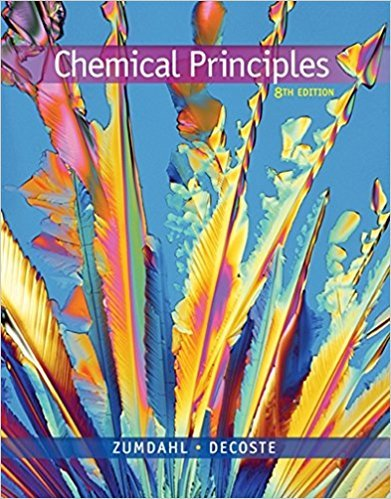×
Get Full Access to Chemical Principles - 8 Edition - Chapter 7 - Problem 7.2
Get Full Access to Chemical Principles - 8 Edition - Chapter 7 - Problem 7.2

×

# What if you lived on a planet identical to earth but forISBN: 9781305581982 176

## Solution for problem 7.2 Chapter 7

Chemical Principles | 8th Edition

• Textbook Solutions
• 2901 Step-by-step solutions solved by professors and subject experts
• Get 24/7 help from StudySoup virtual teaching assistantsChemical Principles | 8th Edition

4 5 1 322 Reviews
23
2
Problem 7.2

What if you lived on a planet identical to earth but for which room temperature was 50C. How would the pH scale be different?

Step-by-Step Solution:
Step 1 of 3

Example 14: ​25 mL ClNH3 0.1 HCl initial pH pKb= 4.75 NH3+HCl­>NH4Cl NH3+H2O­>NH4++OH­ kb=[NH4+][OH­]/[NH3] kb=10^­4.75 ­x +x +x 1.8x10^­5=x^2/0.1 14­2.87=​11.13 0.1 +x +x x=[OH­]=1.34x10^­3 M ­log(1.34x10^­3)= 2.87 ­pH after adding 5mL HCl 0.250(0.10)2.5x10^­3 pH=pka+logNH3/NH4+ ­5.0x10^­4 5x10^­4 ­5x10^­4 pH=9.25+log(0.0667/0.017)=​.84 2.0x10^­3 0.014 ­at equivalence NH3+HCl­>NH4HCl 2.5x10^­3 0.050 mol HCl/ T volume 2.5x10^­3 0 2.5x10^­3 5.6x10^­10=x^2/0.050 x=2.3x10^­6 0 2.5x10^­3 0 NH3 NH4+ H3O+ pH= ­log(0.2.4 )=​ 0 0.050 0 +x 0.050­x +x Add 30 mL HCl HCl= 0.0091 Molar so

Step 2 of 3

Step 3 of 3

##### ISBN: 9781305581982

Chemical Principles was written by and is associated to the ISBN: 9781305581982. This full solution covers the following key subjects: . This expansive textbook survival guide covers 20 chapters, and 2754 solutions. Since the solution to 7.2 from 7 chapter was answered, more than 286 students have viewed the full step-by-step answer. The answer to “What if you lived on a planet identical to earth but for which room temperature was 50C. How would the pH scale be different?” is broken down into a number of easy to follow steps, and 24 words. This textbook survival guide was created for the textbook: Chemical Principles, edition: 8. The full step-by-step solution to problem: 7.2 from chapter: 7 was answered by , our top Chemistry solution expert on 12/23/17, 04:53PM.

Unlock Textbook Solution